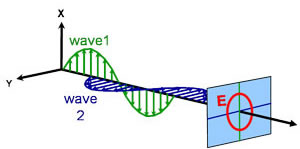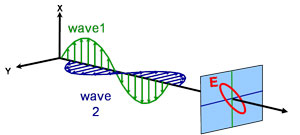# Polarized Light

Light can be described as an electromagnetic wave traveling through space. For purposes of ellipsometry, it is adequate to discuss the waves’s electric field behavior in space and time, also known as polarization. The electric field of a wave is always orthogonal to the propagation direction. Therefore, a wave traveling along the z-direction can be described by its x- and y- components. When the light has completely random orientation and phase, it is considered unpolarized. For ellipsometry, however, we are interested in the kind of electric field that follows a specific path and traces out a distinct shape at any point. This is known as polarized light. When two orthogonal light waves are in-phase, the resulting light will be linearly polarized. The relative amplitudes determine the resulting orientation. If the orthogonal waves are 90° out-of-phase and equal in amplitude, the resultant light is circularly polarized. The most common polarization is “elliptical”, one that combines orthogonal waves of arbitrary amplitude and phase. This is where ellipsometry gets its name.

### Linear### Circular### Elliptical